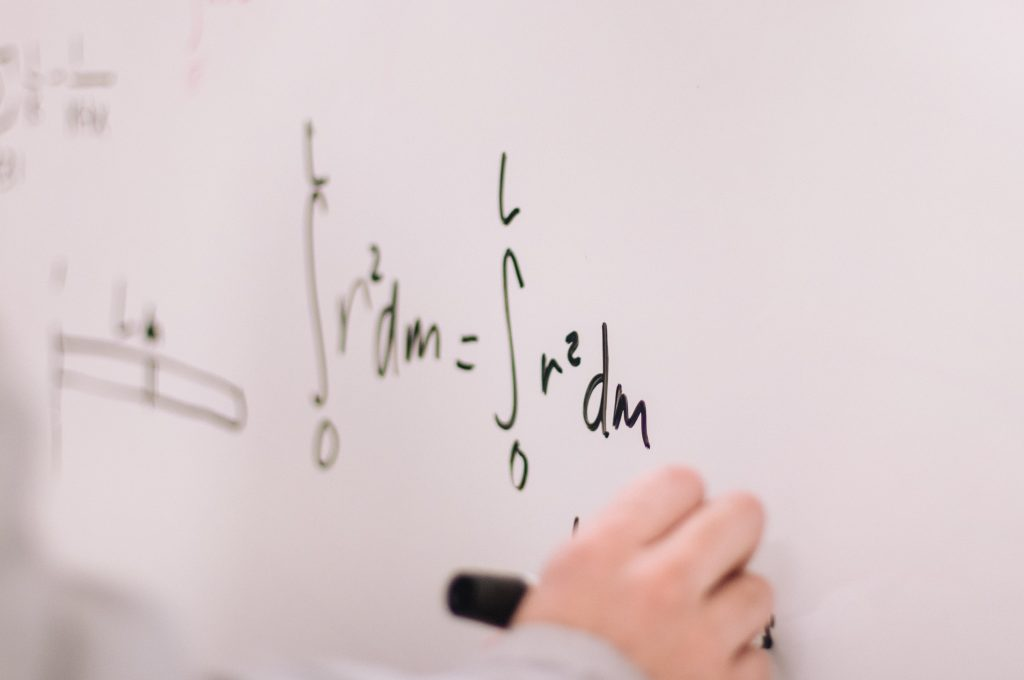## MPM1D

Prerequisite:
NoneThis course enables students to develop an understanding of mathematical concepts related to algebra, analytic geometry, and measurement and geometry through investigation, the effective use of technology, and abstract reasoning. Students will investigate relationships, which they will then generalize as equations of lines, and will determine the connections between different representations of a linear relation. They will also explore relationships that emerge from the measurement of three-dimensional figures and two-dimensional shapes. Students will reason mathematically and communicate their thinking as they solve multi-step problems.

## MPM2D

Prerequisite:This course enables students to broaden their understanding of relationships and extend their problem-solving and algebraic skills through investigation, the effective use of technology, and abstract reasoning. Students will explore quadratic relations and their applications; solve and apply linear systems; verify properties of geometric figures using analytic geometry; and investigate the trigonometry of right and acute triangles. Students will reason mathematically and communicate their thinking as they solve multi-step problems.

## MBF3C0

### Foundations for College Mathematics, Grade 11, College Preparation

Prerequisite:This course enables students to broaden their understanding of mathematics as a problemsolving tool in the real world. Students will extend their understanding of quadratic relations; investigate situations involving exponential growth; solve problems involving compound interest; solve financial problems connected with vehicle ownership; develop their ability to reason by collecting, analysing, and evaluating data involving one variable; connect probability and statistics; and solve problems in geometry and trigonometry. Students will consolidate their mathematical skills as they solve problems and communicate their thinking.

## MCR3U

### Functions, Grade 11, University Preparation

Prerequisite:This course introduces the mathematical concept of the function by extending students’ experiences with linear and quadratic relations. Students will investigate properties of discrete and continuous functions, including trigonometric and exponential functions; represent functions numerically, algebraically, and graphically; solve problems involving applications of functions; investigate inverse functions, and develop facilities in determining equivalent algebraic expressions. Students will reason mathematically and communicate their thinking as they solve multi-step problems.

## MHF4U

Prerequisite:This course extends students’ experience with functions. Students will investigate the properties of polynomial, rational, logarithmic, and trigonometric functions; develop techniques for combining functions; broaden their understanding of rates of change; and develop facilities in applying these concepts and skills. Students will also refine their use of the mathematical processes necessary for success in senior mathematics. This course is intended both for students taking the Calculus and Vectors course as a prerequisite for a university program and for those wishing to consolidate their understanding of mathematics before proceeding to any one of a variety of university programs.

## MCV4U

### Calculus & Vectors Grade 12 – University Preparation

Prerequisite:
Calculus & Vectors, Grade 12- University PreparationsThis course builds on students’ previous experience with functions and their developing understanding of rates of change. Students will solve problems involving geometric and algebraic representations of vectors and representations of lines and planes in threedimensional space; broaden their understanding of rates of change to include the derivatives of polynomial, sinusoidal, exponential, rational, and radical functions; and apply these concepts and skills to the modeling of real-world relationships. Students will also refine their use of the mathematical processes necessary for success in senior mathematics. This course is intended for students who choose to pursue careers in fields such as science, engineering, economics, and some areas of business, including those students who will be required to take a university-level calculus, linear algebra, or physics course.

## MDM4U

### Data Management, Grade 12 – University Preparation

Prerequisite: